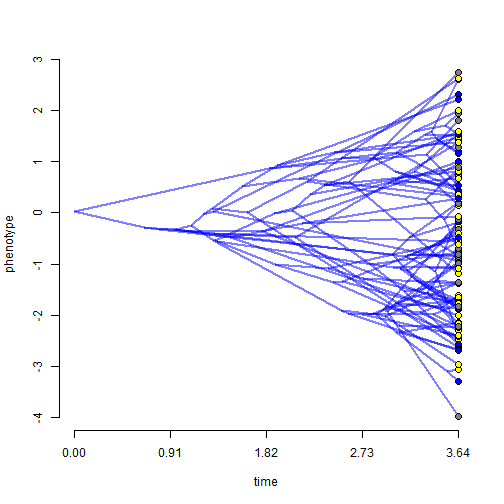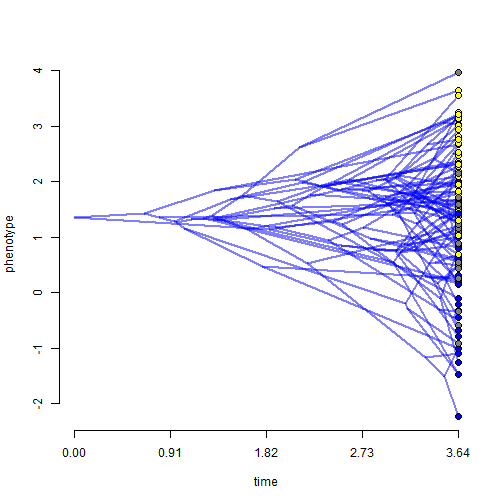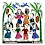## Friday, November 10, 2017

### `print` method for `phylANOVA` (the phylogenetic ANOVA)

A simple method that has long been fairly popular in the phytools package is the function `phylANOVA` that implements Ted Garland et al.'s (1993) simulation-based phylogenetic ANOVA method.

I just pushed a small update that adds a nice print method to the function. Here is an example.

``````library(phytools)
``````

First, our data:

``````phenogram(tree,y1,ftype="off",col=make.transparent("blue",0.5))
tiplabels(pie=to.matrix(x,letters[1:3]),
piecol=colorRampPalette(c("blue", "yellow"))(3),
cex=0.4)
````````````phenogram(tree,y2,ftype="off",col=make.transparent("blue",0.5))
tiplabels(pie=to.matrix(x,letters[1:3]),
piecol=colorRampPalette(c("blue", "yellow"))(3),
cex=0.4)
``````It looks like there might be an interesting pattern in y2, but perhaps not so much in trait y1. In fact, that is precisely what `phylANOVA` will help us quantify:

``````phylANOVA(tree,x,y1)
``````
``````## ANOVA table: Phylogenetic ANOVA
##
## Response: y
##              Sum Sq  Mean Sq  F value Pr(>F)
## x          9.686996 4.843498 2.035856   0.19
## Residual 230.772367 2.379097
##
## P-value based on simulation.
## ---------
##
## Pairwise posthoc test using method = "holm"
##
## Pairwise t-values:
##           a         b        c
## a  0.000000  1.007945 2.015685
## b -1.007945  0.000000 0.869513
## c -2.015685 -0.869513 0.000000
##
## Pairwise corrected P-values:
##       a     b     c
## a 1.000 0.798 0.186
## b 0.798 1.000 0.798
## c 0.186 0.798 1.000
## ---------
``````
``````phylANOVA(tree,x,y2)
``````
``````## ANOVA table: Phylogenetic ANOVA
##
## Response: y
##            Sum Sq   Mean Sq   F value Pr(>F)
## x        79.35828 39.679140 42.007471  0.001
## Residual 91.62362  0.944573
##
## P-value based on simulation.
## ---------
##
## Pairwise posthoc test using method = "holm"
##
## Pairwise t-values:
##          a         b         c
## a 0.000000 -3.225067 -8.964991
## b 3.225067  0.000000 -5.243302
## c 8.964991  5.243302  0.000000
##
## Pairwise corrected P-values:
##       a     b     c
## a 1.000 0.005 0.003
## b 0.005 1.000 0.003
## c 0.003 0.003 1.000
## ---------
``````

Neat. The data were simulated as follows:

``````tree<-pbtree(n=100)
Q<-matrix(c(-2,1,1,
1,-2,1,
1,1,-2),3,3)
rownames(Q)<-colnames(Q)<-letters[1:3]
x<-as.factor(sim.history(tree,Q)\$states)
y1<-fastBM(tree)
y2<-fastBM(tree,sig2=0.5)+as.numeric(x)
``````

1.Hi Liam! How can I plot the legend for the x factor? I tried with add.simmap.legend(colors=x) but it doesnt work...
thank you,
isabel

1.add.simmap.legend(colors=x) will automatically go to the interactive mode, so you have to click where on the plot you would like to add the legend. You can also use legend in base R which is super flexible.

2.Hi Liam, I am a bit new to R and I am trying to learn how to do a phylogenetic ANOVA. I am having a lot of issues figuring out how to format my data correctly so R can read it. Is there somewhere I can go to see the raw data you are using for the x and y variables in this script?

3.Hi Liam,

I haven't been able to find information on what exactly the X-axis represents. I know the default says "time", but when I input my ddRAD tree the axis goes from 0-0.001. Is this something I should be concerned about when also conducting a phyloANOVA? I also tried it with an ultrametic tree thinking the scale would change but it didn't. I was wondering if could shine a light on what the x-axis represents?

thank you,
josh

4.Hi Liam,

I am using the phylANOVA function, but my results are quite weird. The problems are the Pairwise t-values and Pairwise corrected P-values. These two tables have only NA values. Please, would you know to tell me what is happening?

5.Dear Dr. Revell
Is it possible to run phylogenetic MANOVA with this R package? Thanks, Alicia

Note: due to the very large amount of spam, all comments are now automatically submitted for moderation.